# 9.2 Array Objects

An array is a collection of like values ”called elements ”such as an array of colors, an array of strings, or an array of images. Each element of the array is accessed with an index value enclosed in square brackets (see Figure 9.1). An index is also called a subscript. There are two types of index values: a non-negative integer and a string. Arrays indexed by strings are called associative arrays .  In JavaScript, arrays are built-in objects with some added functionality. 

 Creating a multidimensional array (i.e., an array with more than one index) is not officially supported by JavaScript, but can be simulated with some trickery .

 Arrays were introduced in JavaScript 1.1.

##### Figure 9.1. An Array object called color . Index values are in square brackets.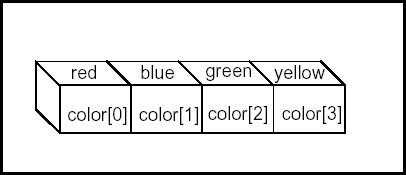#### 9.2.1 Declaring an Array

Like variables , arrays must be declared before they can be used. The new keyword is used to dynamically create the Array object. It calls the Array object's constructor, Array() , to create a new Array object. The size of the array can be passed as an argument to the constructor, but it is not necessary. Values can also be assigned to the array when it is constructed , but this is not required either. Let's examine some ways to create an array.

The following array is called array_name and its size is not specified.

` `
` var array_name = new Array(); `

In the next example, the size or length of the array is passed as an argument to the Array() constructor. The new array has 100 undefined elements.

` `
` var array_name = new Array(100); `

And in the next example, the array is given a list of initial values of any data type:

` `
` var array_name = new Array("red", "green", "yellow", 1 ,2, 3); `

Although you can specify the size of the array when declaring it, it is not required. JavaScript allocates memory as needed to allow the array to shrink and grow on demand. To populate the array, each element is assigned a value. Each element is indexed by either a number or string. If the array index is a number, it starts with 0. JavaScript doesn't care what you store in the array. Any combination of types, such as numbers , strings, Booleans, and so forth, are acceptable. Example 9.1 creates a new Array object called book and assigns strings to each of its elements.

##### Using the new Constructor

To create an Array object, call the Array() constructor with the new keyword and pass information to the constructor if you know the size and/or what elements you want to assign to the array. Values can be added or deleted throughout the program; JavaScript provides a number of methods to manipulate the array (these are listed in "Array Methods " on page 158).

##### Example 9.1
` <html>     <head><title>The Array Object</title>     <h2>An Array of Books</h2>         <script language="JavaScript"> 1  var book = new Array(6)  ;   //  Create an Array object  2           book = "War and Peace"; //  Assign values to its elements  book = "Huckleberry Finn";             book = "The Return of the Native";             book = "A Christmas Carol";             book = "The Yearling";             book = "Exodus";         </script>     </head>     <body bgcolor="lightblue">         <script language="JavaScript">             document.write("<h3>"); 3  for(var i in book)  { 4              document.write("book[" + i + "] "+ book[i]  + "<br>");             }         </script>     </body>     </html> `

EXPLANATION

1. The variable book is assigned a new Array object containing six elements.

2. The first element of the book array is assigned the string "War and Peace" . Array indices start at zero.

3. The special for loop is used to access each of the elements in the book array.

4. Each of the elements of the book array are displayed in the browser. (See Figure 9.2.)

##### Figure 9.2. An array called book displays its elements. Output from Example 9.1.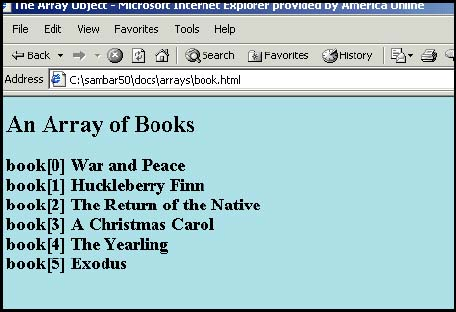##### Populating an Array with a for Loop

Populating an array is the process of assigning values to it. In Example 9.2, the for loop is used to fill an array. The initial value of the index starts at zero; the looping will continue as long as the value of the index is less than the final size of the array.

##### Example 9.2
` <html><head><title>The Array Object</title><body>     <body>     <h2>An Array of Numbers</h2>     <script language="JavaScript"> 1       var years = new Array(10); 2  for(var i=0; i < years.length; i++  ){ 3           years[i]=i + 2000; 4           document.write("years[" + i + "] = "+ years[i]                               + "<br>");         }     </script>     </body>     </html> `

EXPLANATION

1. The Array() constructor is called to create a 10-element array called years .

2. The for loop starts with an initial value of i set to 0, which will be the value of the first index in the array. As long as the value of i is less than the length of the array, the body of the loop will be executed. Each time through the loop, i is incremented by 1.

3. The array is populated here. Each time through the loop, years[i] is assigned the value of i + 2000 .

4. The value of the new array element is displayed for each iteration of the loop. (See Figure 9.3.)

##### Figure 9.3. Output from Example 9.2.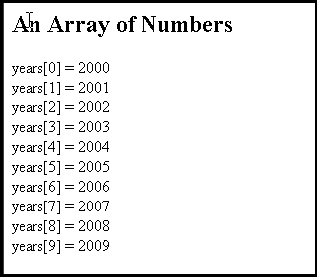##### Creating and Populating an Array Simultaneously

When creating an array, you can populate (assign elements to) it at the same time by passing the value of the elements as arguments to the Array() constructor. Later on, you can add or delete elements as you wish. See Example 9.3.

##### Example 9.3
` <html><head><title>The Array Object</title></head>     <body>     <h2>An Array of Colored Strings</h2>     <script language="JavaScript"> 1  var colors = new Array("red", "green", "blue", "purple"  ); 2       for(var i in colors){ 3  document.write("<font color='"+colors[i]+"'>");  4  document.write("colors[" + i + "] = "+ colors[i]   + "<br>");   }  </script>     </body>     </html> `

EXPLANATION

1. A new array called colors is created and assigned five colors.

2. The special for loop iterates through each element of the colors array, using i as the index into the array.

3. The color of the font is assigned the value of the array element.

4. The value of each element of the colors array is displayed. The color of the font matches the value. (See Figure 9.4.)

##### Figure 9.4. Each string is a different font color. Output from Example 9.3.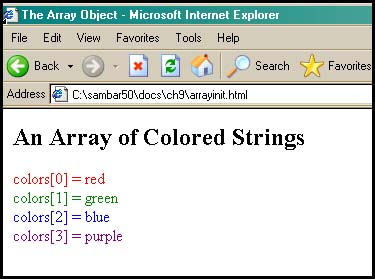##### Associative Arrays

An associative array is an array that uses a string as an index value, instead of a number. There is an association between the index and the value stored at that location. The index is often called a key and the value assigned to it, the value . Key/value pairs are a common way of storing and accessing data. In the following array called states , there is an association between the value of the index, the abbreviation for a state (e.g., "CA" ), and the value stored there ”the name of the state (e.g., "California" ). The special for loop can be used to iterate through the elements of an associative array.

##### Example 9.4
` <html><head><title>Associative Arrays</title></head>     <body>     <h2>An Array Indexed by Strings</h2> 1   <script language="JavaScript"> 2       var states = new Array(); 3       states["CA"] = "California";         states["ME"] = "Maine";         states["MT"] = "Montana"; 4  for( var i in states )  {             document.write("The index is:<em> "+ i );             document.write(".</em> The value is: <em>" + states[i]                            + ".</em><br>");         }     </script>     </body>     </html> `

EXPLANATION

1. The JavaScript program starts here.

2. The Array() constructor is called and returns a new Array object called states .

3. The index into the array element is a string of text, "CA". The value assigned is "California" . Now there is an association between the index and the value.

4. The special for loop is used to iterate through the Array object. The variable, i , represents the index value of the array, and states[i] represents the value found there. It reads: For each index value in the array called states , get the value associated with it. (See Figure 9.5.)

##### Figure 9.5. An associative array.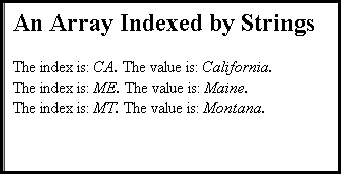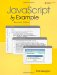JavaScript by Example (2nd Edition)
ISBN: 0137054890
EAN: 2147483647
Year: 2003
Pages: 150
Authors: Ellie Quigley

Similar book on Amazon